## AnSystem in a Magnetic Field*

We will derive the Hamiltonian terms added when an atom is put in a magnetic field in section 20. For now, we can be satisfied with the classical explanation that the circulating current associated with nonzero angular momentum generates a magnetic moment, as does a classical current loop. This magnetic moment has the same interaction as in classical EM,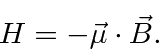For the orbital angular momentum in a normal atom, the magnetic moment is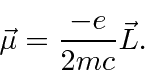For the electron mass, in normal atoms, the magnitude of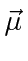is one Bohr magneton,If we choose the direction ofto be thedirection, then the magnetic moment term in the Hamiltonian becomesSo the eigenstates of this magnetic interaction are the eigenstates ofand the energy eigenvalues are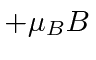,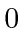, and.

* Example: The energy eigenstates of an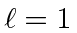system in a B-field.*
* Example: Time development of a state in a B field.*

Jim Branson 2013-04-22